Next: Example 5.2: Motion of an Up: Electric Potential Previous: Worked Examples

## Example 5.1: Charge in a uniform electric field

Question: A charge of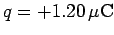is placed in a uniform-directed electric field of magnitude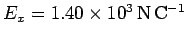. How much work must be performed in order to move the charge a distance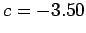cm in the-direction? What is the potential difference between the initial and final positions of the charge? If the electric field is produced by two oppositely charged parallel plates separated by a distance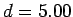cm, what is the potential difference between the plates?

Solution: Let us denote the initial and final positions of the chargeand, respectively. The work which we must perform in order to move the charge fromtois minus the product of the electrostatic force on the charge due to the electric field (since the force we exert on the charge is minus this force) and the distance that the charge moves in the direction of this force [see Eq. (76)]. Thus,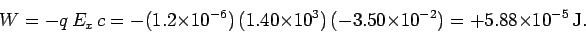Note that the work is positive. This makes sense, because we would have to do real work (i.e., we would lose energy) in order to move a positive charge in the opposite direction to an electric field (i.e., against the direction of the electrostatic force acting on the charge).

The work done on the charge goes to increase its electric potential energy, so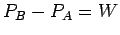. By definition, this increase in potential energy is equal to the product of the potential difference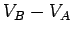between pointsand, and the magnitude of the charge. Thus,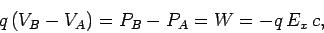giving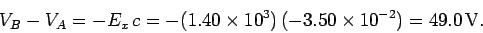Note that the electric field is directed from pointto point, and that the former point is at a higher potential than the latter.

It is clear, from the above formulae, that the magnitude of the potential difference between two points in a uniform electric field is simply the product of the electric field-strength and the distance between the two points (in the direction of the field). Thus, the potential difference between the two metal plates is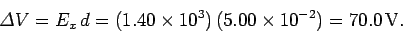If the electric field is directed from plate 1 (the positively charged plate) to plate 2 (the negatively charged plate) then the former plate is at the higher potential.Next: Example 5.2: Motion of an Up: Electric Potential Previous: Worked Examples
Richard Fitzpatrick 2007-07-14